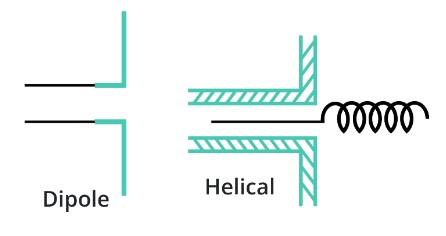# Aperture Antenna Calculator

To use aperture antenna calculator, fill the required input boxes and click the calculate button

Formula:
Eθ = [ ( j*k*a2*E0*e-jkr ) / r ] * Sinφ [ ( J1*k*a*Sinθ) / k*a*Sinθ ]
Eφ = [ ( j*k*a2*E0*e-jkr ) / r ] *(Cosθ*Cosφ) [(J1*k*a*Sinθ) / k*a*Sinθ]

Give Us Feedback

## Antenna Aperture calculator

Antenna Aperture Calculator is a helpful tool designed to help you determine the effective area of a magnetic loop antenna. This tool takes into account various parameters such as magnetic current, half antenna length, wavelength, radius, Z-axis length, and coordinate angle.

## How to Use the Antenna Aperture Calculator?

1. Open the antenna aperture calculator on the web.
2. Input the required parameters in their respective fields:
• Magnetic current (M) in Amperes (A)
• Half antenna length (L) in meters (m)
• Wavelength (λ) in meters (m)
• Radius (R) in meters (m)
• Z-axis length (Z) in meters (m)
• Coordinate angle (θ) in degrees (°)
1. Click on the "Calculate" button to initiate the calculation process.

## What is antenna aperture?

Antenna aperture refers to the effective area of an antenna that captures incoming electromagnetic waves. It is a critical parameter in determining the overall performance of the antenna, as it directly affects the received signal strength and the antenna's ability to transmit and receive signals.## Antenna aperture formula:

The formula to calculate the antenna aperture (A) for a magnetic loop antenna is given:

A = (λ2 * |M| * L) / (4 * π * R * Z * sin(θ))

Where:

• A is the antenna aperture (m2)
• λ is the wavelength (m)
• M is the magnetic current (A)
• L is the half antenna length (m)
• R is the radius of the loop antenna (m)
• Z is the Z-axis length (m)
• θ is the coordinate angle (degrees)

## How to calculate antenna aperture manually?

To manually calculate the antenna aperture (A) for a magnetic loop antenna, follow these steps:

1. Note down the given parameters:
• Magnetic current (M) in Amperes (A)
• Half antenna length (L) in meters (m)
• Wavelength (λ) in meters (m)
• Radius (R) in meters (m)
• Z-axis length (Z) in meters (m)
• Coordinate angle (θ) in degrees (°)
1. Use the formula for calculating the antenna aperture:

A = (λ2 * |M| * L) / (4 * π * R * Z * sin(θ))

1. Substitute the given parameter values into the formula and solve for A.
2. Calculate the sin(θ) value by converting the angle from degrees to radians, as most scientific calculators use radians for trigonometric functions.
3. Perform the calculations, and the result will be the antenna aperture (A) in square meters (m2).

### Example:

Let's consider an example to understand the manual calculation of antenna aperture.

Given parameters:

Magnetic current (M) = 0.01 A

Half antenna length (L) = 0.5 m

Wavelength (λ) = 1 m

Z-axis length (Z) = 0.2 m

Coordinate angle (θ) = 30°

Using the formula, we can calculate the antenna aperture (A):

A = (12 * 0.01 * 0.5) / (4 * π * 0.1 * 0.2 * sin(30°))

A = (0.005) / (0.025 * π * sin(30°))

A ≈ 0.0315 m2

So, the antenna aperture is approximately 0.0315 m2.

## Daily Uses:

Learning the concept of antenna aperture helps in various fields of life as it has a direct impact on signal transmission and reception. A few applications are:

• Wireless communication system: The efficiency of all wireless networks such as WiFi and cellular depends heavily on the antenna aperture.
• Satellite communication systems: It is necessary to enhance antenna aperture in satellite systems for tasks like weather monitoring and television broadcasting.
• Astronomy: Space telescopes use different types of waves such as radio and infrared. So, to capture weak signals, especially from celestial bodies that are quite far away, the antenna aperture has to be amplified.

### Math Tools